# Basic

Basic components for electrical multiphase models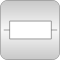# Package Contents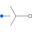Star Star-connection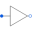Delta Delta (polygon) connection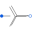MultiStar Star connection of multi phase systems consisting of multiple base systems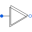MultiDelta Delta (polygon) connection of multi phase systems consisting of multiple base systems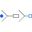MultiStarResistance Resistance connection of star points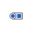PlugToPin_p Connect one (positive) Pin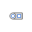PlugToPin_n Connect one (negative) Pin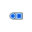PlugToPins_p Connect all (positive) Pins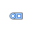PlugToPins_n Connect all (negative) Pins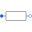Resistor Ideal linear electrical resistorsConductor Ideal linear electrical conductors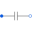Capacitor Ideal linear electrical capacitors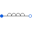Inductor Ideal linear electrical inductors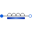SaturatingInductor Simple model of inductors with saturation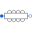MutualInductor Linear mutual inductor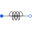ZeroInductor Linear zero sequence inductor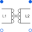Transformer Multiphase Transformer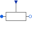VariableResistor Ideal linear electrical resistors with variable resistance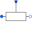VariableConductor Ideal linear electrical conductors with variable conductance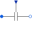VariableCapacitor Ideal linear electrical capacitors with variable capacitance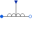VariableInductor Ideal linear electrical inductors with variable inductance

# Information

This information is part of the Modelica Standard Library maintained by the Modelica Association.

This package contains basic analog electrical multiphase components.